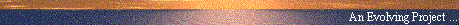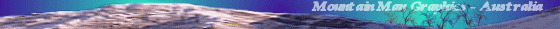## An Overview of the Concept of Attenuation [Pushing] Gravity

#### by Paul Stowe (sci.physics)

##### Web Publication by Mountain Man Graphics, Australia## An Overview of the Concept of Attenuation [Pushing] Gravity

Date: 19 Feb 1999 02:21:07 GMT
From: pstowe@ix.netcom.com(Paul Stowe)
Newsgroups: sci.physics
Subject: An Overview of the Concept of Attenuation [Pushing] Gravity

```

So I'll take up the task of attempting to provide a succinct, but
through briefing of this idea and, as far as I know, its current
correlated status.

The basic idea is that, there is some form of energetic media (some
have proposed light, neutrinos, or other basic particles or wave
dispersion) that is relatively uniform and isotropic throughout space.
This in turn interacts with matter in a fashion as to lose or deposit
some energy into the matter, causing a pressure to be exerted on the
matter.  As illustrated below by vectors A & A':

---
---       ---
--               --
-                   -
-                     -
A'---------------><-----------><------- A
-          C          - P
-                   -
-                 -
--             --
---       ---
---

We see that, if the vectors represent the proposed field's momentum
potential along A & A'.

At point C the vectors are equal and opposite (assuming that the field
was isotropic to begin with, and the body [represented by the sphere]
is of uniform density).  Thus A - A' = 0, and we have no net residual.

However, at point P, A has not encounter the body and is at full
strength, while A', having passed through the body, has endured maximum
attenuation.  Thus A - A' = a, which is a residual vector pointing
towards C.  The magnitude of A' is Ae^-ux where u is a linear
attenuation coefficient, and x is the distance traveled through the
attenuating medium (in this particular case, 2r).  This equation is not
new or radical, it is the general solution to problems of this type,

We can thus re-write A - A' = a to the general form:

a = A(1 - e^-ux)

To obtain the total magnitude of the residual vector we've called a, we
must integrate over all possible paths that involve encountering the
body and arrive at the point of interest (in our case, P).  If we are
dealing with a sphere, this integration becomes independent of the
spatial position of our point of evaluation, as long as it is external
to the body, it is a constant.  The vector a then represents a
'pressure' exerted by the field uniformly on the body.

Now this concept comes in two distinct 'flavors', the strong attenuator
and the weak attenuator.

The weak attenuator is the case where very little impinging radiation
attenuates (as would be the case for neutrinos striking the earth).  In
this case, our problem above becomes a = Aux, since ux is much less
than unity, we can 'linearize' our problem and use the first two terms
of the Taylor expansion [1 - ux].

The strong attenuator is the case where all impinging radiation is
totally attenuated (as would be the case for visible light striking the
earth).  In this case, our problem above becomes a = A since the
exponential becomes e^-oo.

Of course there are the cases where we have neither a 'strong' or
'weak' attenuator (as defined above) but are in the transition zone
between the two.

Now comes the interesting part, when we have two bodies in proximity to
each other as illustrated below:

---                              ---
---       ---                    ---       ---
--               --.            .--               --
-                   - .         .-                   -
-                     -  .     . -                     -
-          x          -    . .   -          y          -
-                     -    . .   -                     -
-                   -   .     .  -                   -
-                 -  .         . -                 -
--             --.             .--             --
---       ---                   .---       ---
---                              ---

the area between them (as indicated by the dotted X region) will have a
reduction in the field pressure due to the mutual attenuation of both
bodies.  This is colloquially called 'shadowing' because it is due to a
shadow cast by each body.  This will then cause the two bodies to move
together due to a 'relative' increase in the pressure on the opposite,
or far sides of each body.  Thus the term, pushing gravity...

Again the form of the equation which will correctly describe this
effect is dependent on whether we are dealing with weak or strong
attenuators.  For weak attenuators it can be shown that the 'apparent'
force generated by the field is:

F = (P[u_sx(rho_x)4pir_x^3/3][u_sy(rho_y)4pir_y^3/3]) / R^2

Where u_s[x/y] is the characteristic 'mass attenuation coefficient' of
the body
rho_[x/y] is the density of the body
r_[x/y] are the radii of the bodies
R is the measured distance between the centers of the bodies
P is the 'maximum' pressure that the field can exert

We'll note here that the linear attenuation coefficient mentioned
earlier is the product of the mass attenuation coefficient (m^2/kg) and
the density of the material (kg/m*3) such that u = u_s(rho).  We'll now
group terms into a more familiar form...

F = (P[u_sx]u_sy)(rho_xV_x)(rho_yV_y) / R^2

Where V is volume = 4pir^3/3

We see that rhoV is mass so we get:

F = (P[u_sx]u_sy)M_xM_y /R^2

Replacing (P[u_sx]u_sy) with a term designated G, we come to a familiar
equation:

F = GM_xM_y/R^2

And the term G does indeed have units of m^3/kg-sec^2.

For strong attenuators the force generated simply is:

F = P(pi[r_x^2])(pi[r_y^2])/R^2

Which is NOT the Newtonian force equation, and is in no way related to
the mass of the bodies.

We note that, for this concept to work as described, there IS a
transfer of energy from the field into the bodies.  For weak
attenuators this transfer is directly proportional to the pressure
generated due to attenuation.
Thus the rate of energy transfer (power Q) is:

Q = Q_in - Q_out

And Q_out is (Q_in)e^-ux

Working this through for the weak solution leads to a very simple
equation describing the power transfer due to attenuation, when put in
terms of per unit (q) area we get:

q = kM/r

Where M and r are the mass and radius of the body, and k is:

(q_max)2G/c^2 (m/sec^3)

The strong solution should be obvious...

Therefore, when in equilibrium, the amount of thermal power radiated
back into space should be AT LEAST q defined above, since in this case,
Q_in from the field should equal Q_out in the form of black body
radiation.  Therefore the above equation for the thermal output q
becomes:

q = kM/r(1 - e^-Ht)

where H is called the thermal inertia coefficient, and is:

H = (UA/MC_p)

U is the overall heat transfer coefficient
A is the exposed surface area
C_p is the heat capacity at constant pressure
And t is the total age of the body in seconds…

I have 'normalized' k to the moon's anomalous output of 0.01 and
determined its value to be ~ 2.4E-19.  If one is so inclined, they can
check this equation's predictive ability against the known thermal
emissions of all the other known net thermal emission of the planetary
bodies.  Here's what I get:

Body     Mass     Radius     Net Flux   Predicted Flux   Difference

Moon    7.4E+22  1.74E+06      .01          .01              -
Earth   6.0E+24  6.37E+06      .06          .042*            ?
Jupiter 1.9E+27  7.18E+07     6.60         6.40             3.4%
Saturn  5.6E+26  6.03E+07     2.30         2.20             2.7%
Uranus  8.6E+25  2.67E+07      .76          .78            -2.1%
Neptune 1.0E+26  2.49E+07      .76          .97             -27.3%

* the earth CAN NOT BE in thermal equilibrium thus the output will be
below the equilibrium value

It is also obvious that if a body were moving through the field, such
that the impinging flux is no longer isotropic, there will be a
differential pressure generated between the leading and trailing sides
of the body.  This in normal hydro-dynamical models creates a drag
force.  This is one of Feynman's objections stated in Volume 1 of 'The
Lectures' pages 7-9 & 7-10.  The magnitude of this force can be
calculated IF one 'assumes' that the attenuation generated by such
movement is similar to the impingement effect described above.
However, this probably isn't a valid assumption.  Again using the
'hydro-dynamical' model as a guide, a fluidic medium will a
lways follow the path of LEAST resistance.  In the case of a stationary
body, this path is through it (or not at all), for said moving body, in
is either through it or 'around' it which ever is easier... and around
it will be the easier path.  As such, the magnitude of the drag will be
greatly reduced, but probably NOT eliminated entirely.

There has been much ado about the fact that in GR there are no forces
acting on bodies even though they will free-fall (accelerate)
describing a non-linear path.  It is to be noted that in the weak
solution shown above, any body responding to an acceleration field does
so uniformly, without any differential stresses such that there is no
way to detect this acceleration from the body's perspective.  We note
that the acceleration that a material body will experience in such a
field results in the standard F=ma equation for force, and acceleration
gives a = F/m and a = GM/R^2

Therefore, the acceleration of any matter body to this type of field
current is not dependent on the mass of the body. Any matter body
responds to a field current in the same manner (regardless of its
mass).  The important concept here is that field current creates an
acceleration independent of mass, and the resulting "force" is only a
byproduct of this acceleration.  This is the principle of equivalence.

So, to summarize the evidence supporting the attenuation gravity model
we have:

1. The gravitational constant is measured to have the same units as
those predicted by this model
2. The weak solution case matches Newton's equation
2. The Newtonian equation is the weak solution limit of the model and
IS also the weak solution limit to the GR mathematical model
3. RFD, gravitational red-shift and other elements predicted in GR are
inherently present in physical mechanism of the model
4. The induction heating predicted matches astronomical observations

All substantive comments and questions are welcomed...

Paul Stowe
E-Mail: pstowe@ix.netcom.com(Paul Stowe)

```Usenet Index | Mountain Man Graphics | E-Mail